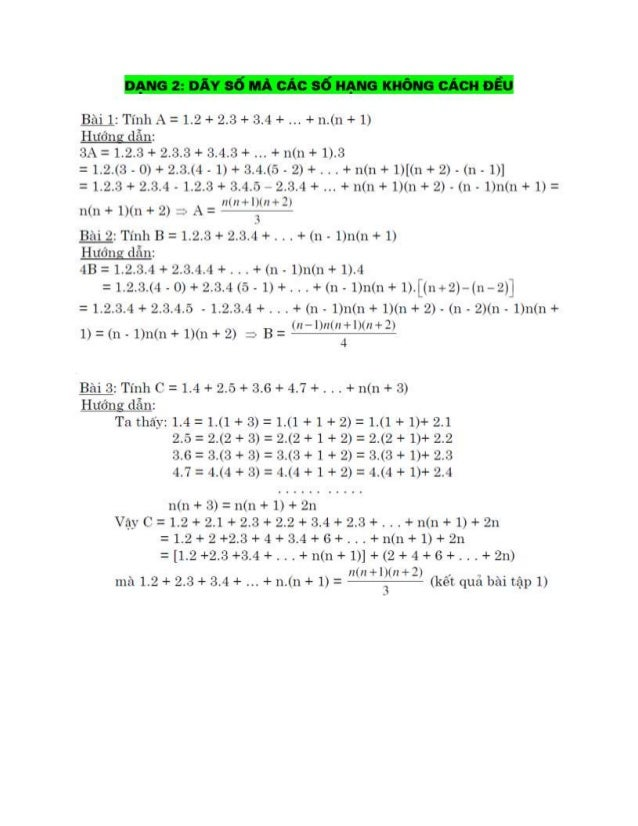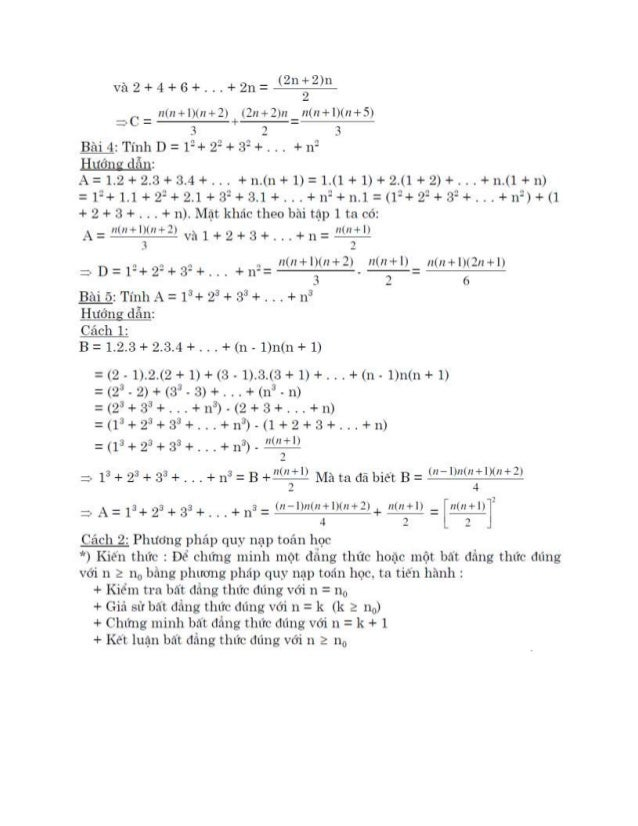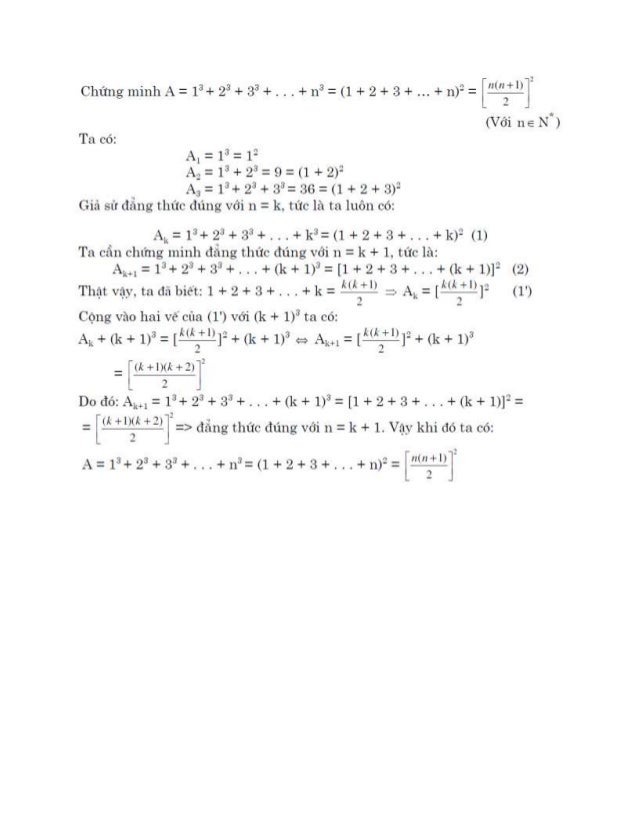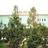Successfully reported this slideshow.Upcoming SlideShare
×

ofUpcoming SlideShare
MỘT SỐ BÀI TOÁN VỀ DÃY SỐ - SỐ TRANG
Next

3

Share

# Dãy số mà các số hạng không cách đều

Luyện thi phần dãy số mà các số hạng không cách đều, ôn thi hsg trung học cơ sở.

See all

See all

### Dãy số mà các số hạng không cách đều

1. 1. DẠNG Z: DỈY SỂMẨ CỈC SỂHẠNG KHỂNG CỈCHĨỈÐỈU Bâìi 1: Tílìlì .- = 1.12 + 12.23 + ấẫ.^l + + lì.(Iì +1) HLĨỞIIƯ cĩẳxlz 3.- = 1.Z2..^3 + 22.23.23 + Iẳ.4.iẳ + + l1(II + 1).23 =1.22.(.`3- 0) + ỉZ.I3.(-1 ~ 1) + I3.4.(õ - 22) + . . . + l1(l1 +1)l(I1 + 2)~(1ì -1)] =1.2.23+ 2.23.4 - 1.2.13 + Ỉ3.4.õ - 2.I3.4 + + l1(I1 +1)(I1+ 2) - (11 - 1)Iì(l1+ 1) = I:IIl+lltI!+2› lì(Iì +1)(Iì + 2) *IA .- = Bẫìí 2: Tílìll B = 1.12.-'3 + 2.I3.Ẩ + . . . + (11 - 1)ll(l1+ 1) Hướlìơ tlẫlìz ì 4B ='1.2.ầ3.^1+ :ẩ.13.4.4 + . . . + (11 - 1)Iì(Iì +1).4 7 7 =1.2.ỉ3.(4 - 0) + 22.23.-1 (Ỗ - 1) + . . .+ (lì ~1)1ì(1ì + I).Ị(ll+:Z)-(11-2)l =1.2.ầỗ.~l+ 2.23.-1.5 - 1.2.23.-1 + . . . + (11 - 1)Iì(Iì+1)(lì + 2) - (lì - `2)(1ì - 1)1ì(lì + 1) T- (II -1)I1(I1 + 1)<I1+ 2) :v B= m4Wỉị Bŕli23:Tíl111 C =1.-1 + 2.5 + ấ3.6 + -1.7 + . . . + I1(I1 + 23) IIlr‹Ĩíl1Ơ clẫlìt Ta Ì11Íìdỹ'2 1.4 =1.(1+23)= 1.(1+1+ 2) = 1.(1+1)+ 2.1 12.õ = :2.(2 + 23) = 2.(2 +1+ 2) = 2.(12 + 1)+ 2.2 23.6 : ẩ3.(:3 + cỗ) `~` :ẵ.(t3 + 1 + 2) = 23.(:3 +1)-F 2.23 -1.7 = -1.(-1 + 23) T- -1.(~1 + 1 + 2) = -1.(-1 + 1)+ 12.-1 Iì(lì + 33) = 1ì(1ì + 1) 4' 211 “`:'__' C` = 1.2 + 2.1 + 2.23 + 2.2 + 23.4 + 2.3 + . . . + I1(l1 + 1) + 211 =1.2+2+2.ễ3+4+Z3.4+6+...+11(11+ 1)+211 = [1.2+2.:3+:3.-1+. . .+1ì<Iì+ 1)] +(2+ ^I+6+. . .+'.2lì) lI(Il+lDIIl°2) 3 11151 1.12 + 2.23 + ẵầ.4 + + lì.(I1 + 1) = (kẽμt rịuĩi lìàì t2^_ì}) 1)
2. 2. Yà2+í4+6+...+2lì= llírt+l)(II+2l l2Il+2)II Ill.Il+l)(lì+5) :sC=ì+ị:Ĩ 3 2 3 Bài `1ZTĨIìl] D=1S+ 2ỉ+ì33+. .. +I1c Hltởlìg dẳlìz A=1.2+2.3+3.4+...+n.(11+1)=1.(1+1)+2.(1+2)+...+1l.(1-F11) =1=+1.1+2=+2.1+:3=+:3.1+...+11=+11.1=(1=+2°+:3=+...+x1=>+(1 + 2 + 23 + . . . +11). Níật klìảc tlìeo bài Ìậl) 1 ta CÓ: IlIIl+l} 2 , 7 A=ẸFà1+2+ì3+...+I1= D 1n+ ).,+ 3,+ + n Il(Il+l)(Il+2) ll(Il+l) ll(Il+l)‹2Il+I) :› =- 2.- 2- 11-=-Ì- -Ì-Ệ Bài Ỗ: TíIII1.~= 13+ 23 + 33+ . . , +11'3 HƯỐl1g dẳlìz Cềẫcìì 1: B = 1.2.33 + 2.33.4 + . . . + (11 - 1)I1(Iì + 1) 3 2 Ó =(2ñ°1).2.(2+1)+(3- 1).23.(8+1)+. ..+(n- 1)l1(n+1) =(2°-2)+(3J~3)+...+(I1“j-11) =Í2ằ+ẵẵ3+.Ĩ.'Ỳ'I13)-(Ỉẵ'Ổ'Ểẵ`Ỗ”...+lì) =(1°`+23+8J+...+n“)-(1+2+8+...+11) =(13+2a+83+I Ợ'+na). Il(IIì+ÍÌ :. 13 + zề* + f3°` + . . . + 11°` = B +1'-'.Ĩ'1+" 1Ià ta đã biê't B = íl“lĩ"l-í'ỊĨDíỈ'I3 , .. .. ..Ì V " :_LA=13+ 234_ 23s+Q . . +lìo= (ll l)IIIII4+l)ỈII -)+IlÍlIw+lI=lIIÍII1†ỈIJ Cáclì 2: Phươlìg plìáỵì qus' Iìạp toáxì học `k) Kìểμll t.lltì'c 2 Để cllthlg lllílìh ulột cĩấấlìg tìlúc lloẽịc Illột bãt ttãẫllg [`11tĨC dtìllg 'ớ`1 11 2 110 lìàlìg Ị)11trơl1g Ị)11áp c;u_X' l1ạỊJ toáll Iìọc. ta t.ỉẻVx1 11àI111 : + Kìểlìì t1`a bãt đằlìg tlìlĩc đtìlìg 'ỞÌ II = 11° + Giả Sfrbđt đẳllg tlllĩc dtìllg 'ớì II = k (k Z 110) + Clllhìg 11111111 ba't dẳlĩg t.l1tĩC đtìllg 'ỞỈ lì = k + 1 + Két luận bált dẳng tlltĩc đlìlĩg với 11 2 110
3. 3. Cllứlìg lìììlìlì A = 13+ 23 + 233 +. . . + 113 = (1 + 2 + Z3 + + I])2 = ífvęĩìl-Jẫvị-ì-Ì (Vớí IIE N*) Ta có: A,=13= 1= .Ầ,= 13+23=9=(1 +2)= A3: 13+ 23+:33=36=(1 +2+:3)= Giả str đẳlìg thức đúlìg với 11 = 1;. tức là ta Iuôlì có: Ak=1”+ę3+233+...+k3:(1+2+:3+...+k)= (1) Ta Cẩlì chlĩtìg lnính đàng tlìửc đúlìg với n = k + 1. tvức là: Ak+1=13+23+83+..,+(k+1)3=[1+2+3+...+(k+1)12 (2) Ả(Ắ+l| 'I`11ật wvậ;v. t.a đã biê't.: 1 + 2 + 3 +. . . + k - em-_=m :u Ak = [Ỗị+-ỂÌ1= (1') Cọlĩg 'à0 llaí Vè' cíla (1') với (k + 1)3 t.a Có: Ả(Ả [uđẤ +lÌJỈ+(k+1)3=AẦẬl=[ +lỪJỀ+(k+1)3 'Ì Ì ”‹A +l)(k +2)`jl I 3 I D0đỏ:.Ẩk†,= 13+ẩẵ+8`”+...+(1‹+1)3=[1+2+3+...+(k+ 1)]== = => đẳlìg tlìức đúng 'ớỈ 11 = k + 1. Vậs' klìỉ đó ta có: a^xμ+‹k+1›3=I A=1=*`+2'°`+:33+...+n=*=(1+2+:3+...+11)==í IItlt+l):l1
4. 4. Bài G: Bçñi 6 (t1`t111g 223/SGK toálì 7- tậịì 1) BỈét` 1`à11g 1"`+ 22+ ề3°“`+ . . . + 10: = 2385. đỏ 9111 tíllh Iìlìanlì đtrợc t.Ổ11g S=2°+4°+6°+...+20” Httớlìg dấlìz Ta có: s= :7.=+ 4=+ 6=+ . . . + 2 ==(2.1)= + (2.2)= + . . . + (2.10)= = 1`-`.2= + 2=.2=+ 2=.3Ề +. . .+ 2=.10° 2 = *l.ề38õ = 1ỗ40. Nltrìrl .t-cắt: Néu ‹Iạt P = 1= + 2= + :3=+ . . . + 10-` t`l1`lt.ả1 có: S = 4.P. Do đó. 11ẻ'Ll C110 S tlìì t.a sẽ tílìlì dlrợc P 'à Iìglrợc lại. Tớlìg quát lìóa ta CÓ: P : 1=+ 2=+ :32+ . . . + 112 = (tlleo ké't cịuzì bẫli tajìỊ› 4 Ở t1'èII) Iẫĩlí dó S = 22 + 42 + 62 + . . . + (2I1)2 dtrợcf tílllĩ ttrơìlg tt_r lìlltt bài Ỉ`I'ềlì. ttl Có: S = (2.1)=+ (2.2)= +. . .+ (2.11)== 4.(1=+ 2=+ 3=+ . . . + 11=) = _ -ŨIIíll'Ỉ"lÌÍẶ`ỈI!`ÝịlụÌ _ ẫ'_'ẵ7“Ỷ'l)_í_2_"`Ỷ'lỲ Ỏ .Ấ Il(II+l) Ù Còlìz P 3 13+ 2`3+ 23°`+ .. .+ I1°` = Í Ì . (theo kÉ^t quả bàitập -5 Ờ t1'c"1ì) Ta tílìlì S = :2'3+ 43 + 63 + . . .+ (ẺIIẦSIIIIII Salt: S = (2.1)J 4' (ĩ2.I2)°` “F (2.23)s + . . . + (2.11)J = 8.(1J+ 23+ 33+ .. . + 113) => S = 8P. Vgì}° ta Có: _llllI_+l)q2 _ S.ll2(lI+l)2 S=23+ 4134' 63+...+í2l1)3= 8-I ~ì**I * 1 ù"-'2II2ÍII'Ỷ'ỈÌ: Bài 7: a)Tí1ìlì A-“2 12 + 23° + Ỗ: . . + (ẩlì -1)2 b) Tíllh B = 13+ B3+ 53 +. . . + (211-1)3 Hướlìơ clẫlìz
5. 5. a) T1100 ket q11:` ÌJàì 4 Ở t`1`Ềl1. ta CÓ: 2lI(2Il+lDt4/I Ơ I) _› lIl2/I+ lì(-lII+ ll I`I Ờ 3 1.'-'+ 2°2+ 23ề`+ . . . + (211)2= ẵl ttl Ỉ`11ẩI._'2 .1 1"-`+:ịấ +:Ĩì=+...+‹211-1)= = 1=+2“-'+:3=+...+<211-1)=+(211)=-[2=+J.=+6=+...+t211)=] IlI2Il+I)[4Il+IJ 2IIIIl+l)IÌII+lD_ IlÍ4IlJ“lÌ - ,`s _ _ĩ _ầ b) Ta CÓ: 1ẵ+ :3"“+ .5*›“+ ...+ (2ỵỵ-1)*ì: 1*+ 2J+ 23-B"+ . . . + (211)"“- I23+ 4`*+ 6“*+. . . + (211)`ì] ấl) ‹ll_ll1g kẻ`T cltlà l)â`ìÌ tf_ìỊ,ì số C3 t1`èIì Ĩỉì tĩtắ: 13+ 2'ì+ 33S+ . . . + (2Iì)`Ệ = lì2(211 +1)ì. "zịì_X': B = 1a+ I3'3+ ả3'3 + . . . + (211-1)3=112(2I1 + 1)2 - 2I12(l1 + 1): `-' Z311" - 112 BẳỈ8ZTÍ]1Ì1S1=1+2+Ễg+2Vj+...+2`Ảj HlÌ‹311f,v tlẳllz Ctícll 1: 2+2ì+2d+...+2U3 (1) . .I +2°=+:2”3`+,.,+'2*ẵ“3+2'5" (2) T1'ỉrt1`nìg 'ứ Cl`ltì 2) C110 (1) tậì Ctẫz 2+2=+2`°`+...+2'Ầẳ+2°*-(1+2+2=+2“+. ..+2*”°*`) = 2C"ì - 1. Hz_' S1= 26* - 1 Ờácll 2.' Tz1có:S1=1+2+2=+2`3+...+:ZỆS=1+2(1+2+2“ =1+2(S,-2'ìVj)=1+2S1-2'““ :v SL=L `-1 Bài 9: Tílìlì gíá t1`ị Cíla bìếu tlìlĩc S = 1 +33 + 232 +1 " Httrĩxìơ (lẫllz Ũtíclt 1: 1âj› clịllìg Czìclì Iẵlllì Cl`lzì l):`ìi tzịỵì t1`êI1 Tzì cks: 238 = 23 + :3= + 233 + ,. . + :ẵ›“=r'*"* (2) T1'ír tínìg "t“` Cl`Ia (2) C110 (1) ta dlltỹcỉ :3S - S = (23 + :3*` + 23°” +. . . + :3='”°*> - (1 +:3 + 232 + :3*3 +. . . + :3=“”l`°) ì`ll!l "V “ ~l Ha}': 2h J Cồ ĩẫ2"l`μl ~ Ì :- S = VĨ Ì
6. 6. Crích 2: Ttrcnìg tịr lĩlĩlr Caìcll 2 Cílzì ljẵli Ỉ`1'Ẻl1Z T11 có: S = 1 + 3(1 +23 + BẾ + :3B3 + . . . + :3`*“-S'^Ấ"Ầ') = 1 + 3(S - :3=í'°iJ) = 1 + :3S - :3=`í`°l WẶIII _I Í-2S=ì,2fĨ'°l-1:-S=" `^`) Tóllg qllzìt Iloá 111 L'L8: Slì = 1 + (Ị + (12 + (13 +. . . + L1” (1) Klìì dó ta Có: C'ácÌl 1: qSn = q + q" + cỊ"` +. . . + q“`Ỷl (2) tỊt`ll -Ì T1`Ìrtír11g 'è cŕlaì (2) C110 (1) lil Có: (cl - 1)S = cỊ"" - 1 :v S = I ‹Ị- Ũá('Ìỉ 2: Sll = 1 + cỊ(1 + q + (lì + (l`3 + . . . -Ố' cỊ'1”l) Z 1 + q(Sl1- qu) '-` 1 + (1811 - cỊ“`L :~ ‹ỊS,, - Sn = ‹j1"1 - 1 1l1ì_': s,ỵ(c1- 1) =c-1I`** ` 1 :. S = 'lữ` Bẫìi 10: C110 : = 1 + 2 + :Z"` + 2`3 + . . . + 29: B = Ỗ.Ầẵ5. So .SRÍIIII .-8 'Ỉì B Hướlìơ clẳlìz Taìcó:xx=l+2+2=+2'*+...+2° (1) 2.w=2+2=+2'a+...+2°+2*° (2) T1`[r tínìg 'C Ct`la (Z2) C110 (1) ta Có: :2.ằ‹.ằ= (2+2=+2'3+...+29+2l“')-<1+2+2=+23+..,+2°> =21”- 1l1ỂI_'.Ốt= 1 c'ò11: B = ;3.2~* = (2= + 1›.2"` = 21° + 2Ề` Bẫlí 11: Tílììì giá t1'ị ‹'1`l‹'l bỈL“"11 tlìlĩc S = 1 + 2.6 + 23.62 + 4.6`” + . . . + 100.6ç'ç° (1) I¶Lt‹ỈỉI1Ơ tĩẳllz Tel có: GS = 6 + 2.6= + 25.63 + + 9E).6ç'9+ 1(ì0.61('c' ‹2> T1`Ỉr tínìg `ęB Cỉla (2) C110 (1) ta dlrợcz aõs = 6- 2.6 + (2.63 - :3.‹3=› + ‹:3.G3 ~ «l.‹3'Ĩ) + . . . + <99.G°° - 10(ì.‹3°”) + +100.6"""-1=100.6=‹`‹'- 1 - (G+ 63 + Gìì + . . . + 6Ể'°) (*) Dzịt S'=G+G"°+6'a+. ..+6Ế"í' :- 6S'=6=+G3+...+6°Ế'+6ì*'°' :r :- S' = 6I.Ib-6 5 s3S = 100.6l°“*- 1 - tBl1a_' °ỂìO (*) ta có: ‹1""- 6 _ 4l›t›,h"" +1 Ễ 3 4t›l›.t›"“` v I `< :~SZ
•#### nhNguyen8

Jul. 3, 2020
•#### duyha524

Jul. 8, 2019
•#### HngV110

Aug. 26, 2016

Total views

21,773

On Slideshare

0

From embeds

0

Number of embeds

13,895

0

Shares

0